Chapter 7 Chemical Reactions Experiencing Chemical Change chemical

• Slides: 68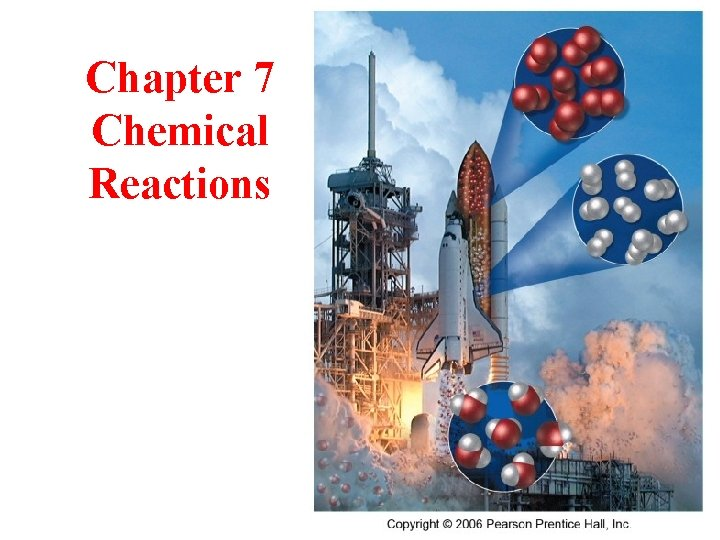Chapter 7 Chemical ReactionsExperiencing Chemical Change • chemical reactions are happening both around you and in you all the time • some are very simple, others are complex ü in terms of the pieces – even the simple ones have a lot of interesting principles to learn from • chemical reactions involve changes in the structures of the molecules, and many times we can experience the effects of those changes • What are some examples of chemical reactions you experience? 2Chemical Reactions • Reactions involve chemical changes in matter resulting in new substances • Reactions involve rearrangement and exchange of atoms to produce new molecules üElements are not transmuted during a reaction Reactants Products 3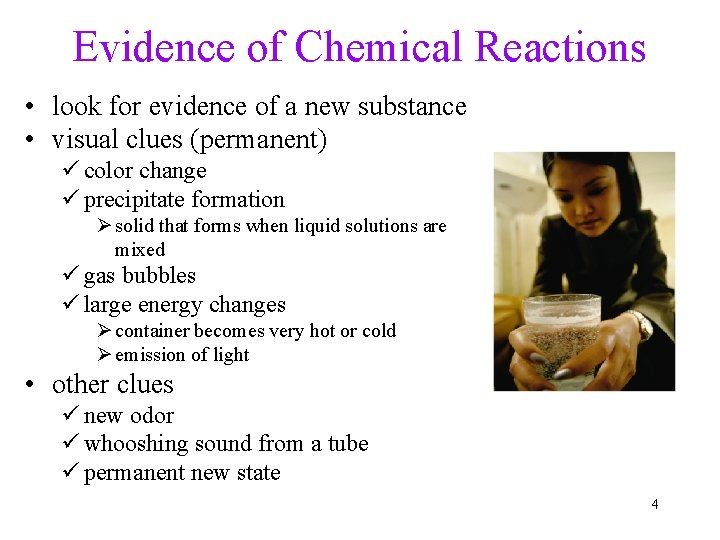Evidence of Chemical Reactions • look for evidence of a new substance • visual clues (permanent) ü color change ü precipitate formation Ø solid that forms when liquid solutions are mixed ü gas bubbles ü large energy changes Ø container becomes very hot or cold Ø emission of light • other clues ü new odor ü whooshing sound from a tube ü permanent new state 4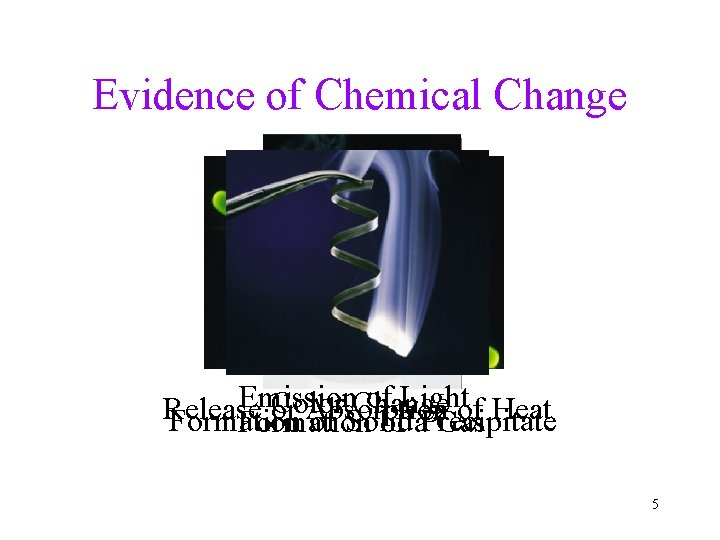Evidence of Chemical Change Emission of Lightof Heat Color Change Release or Absorption Formation of Solid Formation of a. Precipitate Gas 5Evidence of Chemical Change • in order to be absolutely sure that a chemical reaction has taken place, you need to go down to the molecular level and analyze the structures of the molecules at the beginning and end Is boiling water a chemical change? 6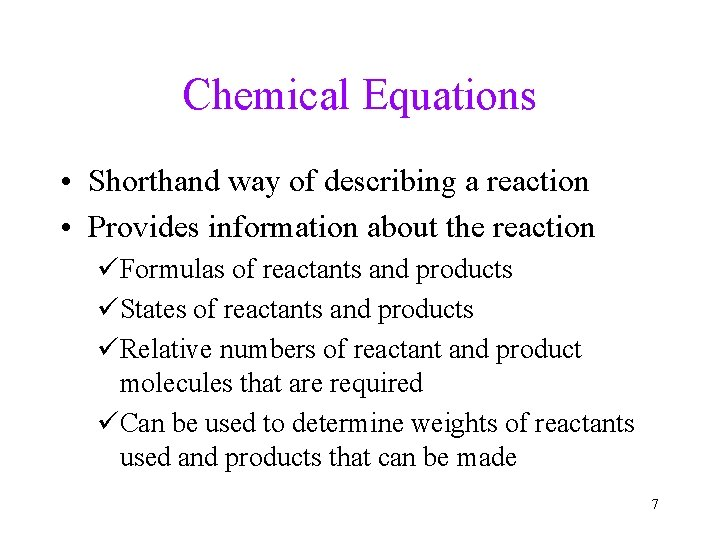Chemical Equations • Shorthand way of describing a reaction • Provides information about the reaction üFormulas of reactants and products üStates of reactants and products üRelative numbers of reactant and product molecules that are required üCan be used to determine weights of reactants used and products that can be made 7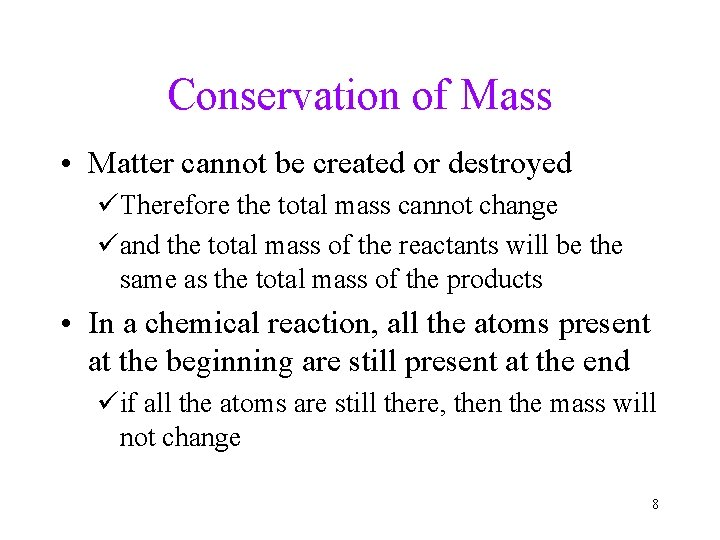Conservation of Mass • Matter cannot be created or destroyed üTherefore the total mass cannot change üand the total mass of the reactants will be the same as the total mass of the products • In a chemical reaction, all the atoms present at the beginning are still present at the end üif all the atoms are still there, then the mass will not change 8The Combustion of Methane • methane gas burns to produce carbon dioxide gas and gaseous water üwhenever something burns it combines with O 2(g) 9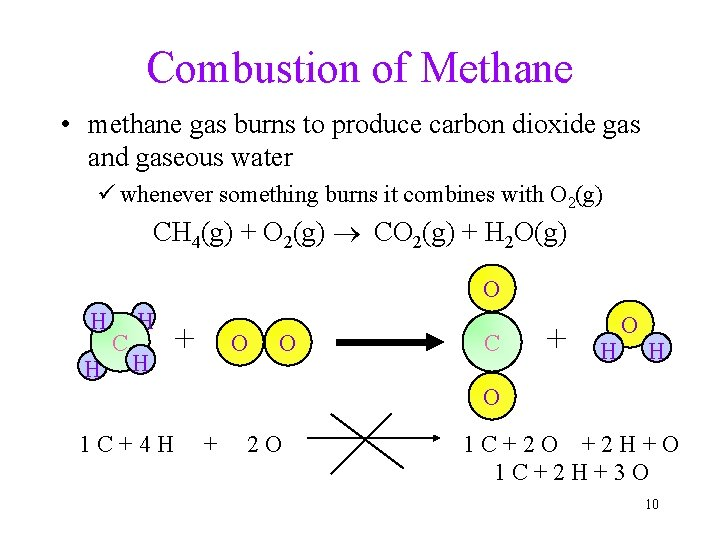Combustion of Methane • methane gas burns to produce carbon dioxide gas and gaseous water ü whenever something burns it combines with O 2(g) CH 4(g) + O 2(g) CO 2(g) + H 2 O(g) O H H C H H + O O C + H O 1 C+4 H + 2 O 1 C+2 O +2 H+O 1 C+2 H+3 O 10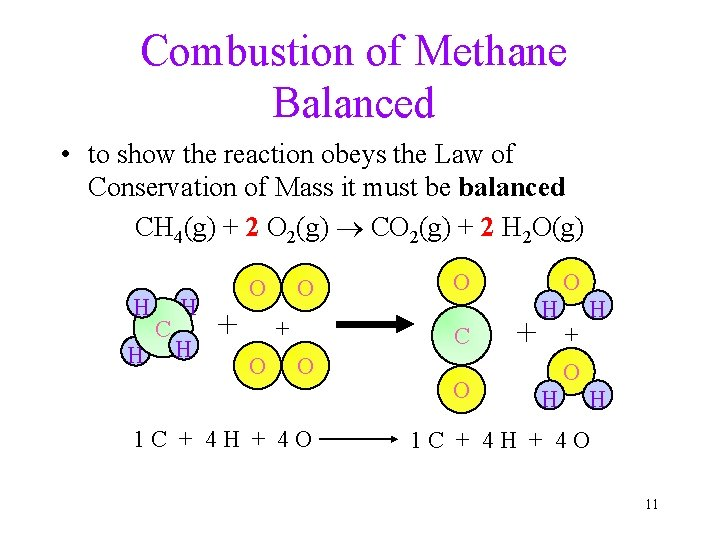Combustion of Methane Balanced • to show the reaction obeys the Law of Conservation of Mass it must be balanced CH 4(g) + 2 O 2(g) CO 2(g) + 2 H 2 O(g) H H C H H O + O O C O 1 C + 4 H + 4 O O + H H O + O H H 1 C + 4 H + 4 O 11Chemical Equations CH 4(g) + 2 O 2(g) CO 2(g) + 2 H 2 O(g) • CH 4 and O 2 are the reactants, and CO 2 and H 2 O are the products • the (g) after the formulas tells us the state of the chemical • the number in front of each substance tells us the numbers of those molecules in the reaction ü called the coefficients 12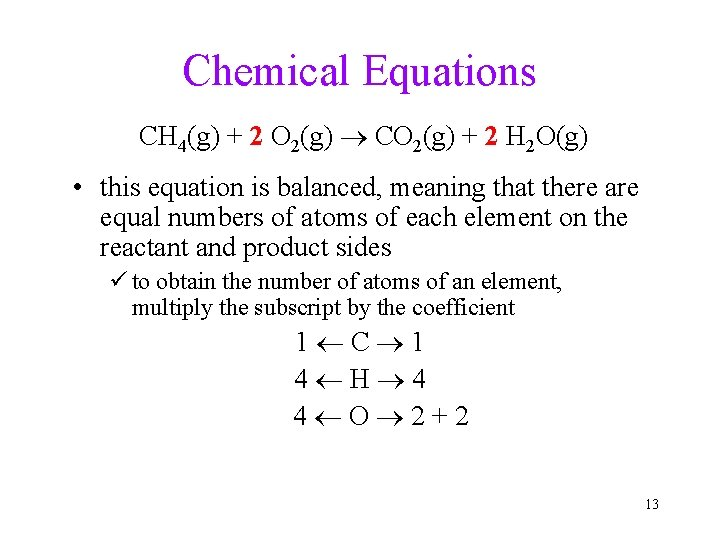Chemical Equations CH 4(g) + 2 O 2(g) CO 2(g) + 2 H 2 O(g) • this equation is balanced, meaning that there are equal numbers of atoms of each element on the reactant and product sides ü to obtain the number of atoms of an element, multiply the subscript by the coefficient 1 C 1 4 H 4 4 O 2+2 13Symbols Used in Equations • symbols used to indicate state after chemical ü(g) = gas; (l) = liquid; (s) = solid ü(aq) = aqueous = dissolved in water • energy symbols used above the arrow for decomposition reactions ü D = heat ü hn = light üshock = mechanical üelec = electrical 14Writing Balanced Chemical Equations 1. Write a skeletal equation by writing the formula of each reactant and product 2. Count the number of atoms of each element on each side of the equation ü polyatomic ions may often be counted as if they are one “element” 3. Pick an element to balance ü if an element is found in only one compound on both sides, balance it first ü leave elements that are free elements somewhere in the equation until last 15Writing Balanced Chemical Equations 4. Find the Least Common Multiple of the number of atoms on each side ü the LCM of 3 and 2 is 6 5. Multiply each count by a factor to make it equal to the LCM 6. Use this factor as a coefficient in the equation ü if there is already a coefficient there, multiply it by the factor ü it must go in front of entire molecules, not between atoms within a molecule 7. Recount and Repeat until Balanced 16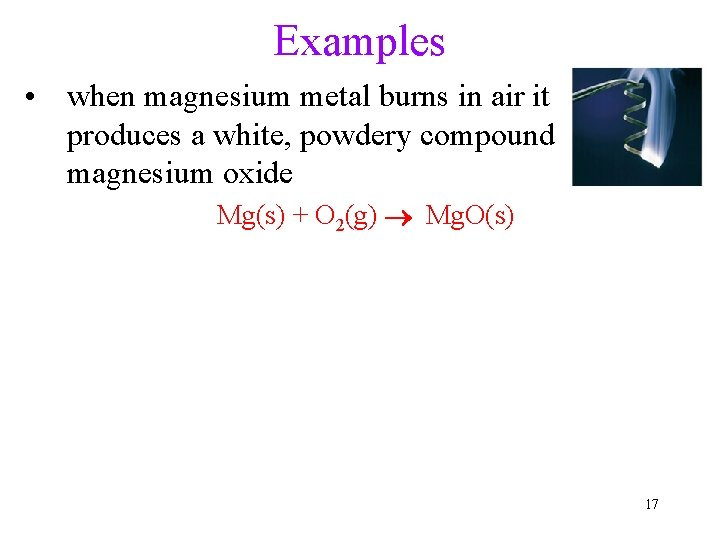Examples • when magnesium metal burns in air it produces a white, powdery compound magnesium oxide Mg(s) + O 2(g) Mg. O(s) 17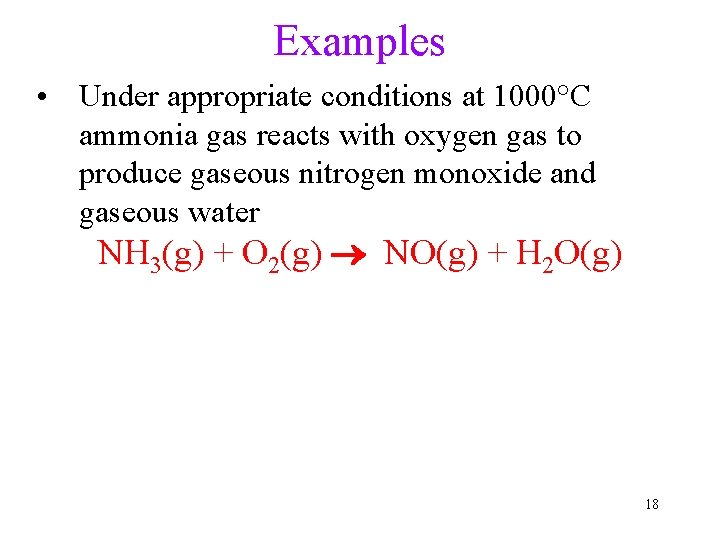Examples • Under appropriate conditions at 1000°C ammonia gas reacts with oxygen gas to produce gaseous nitrogen monoxide and gaseous water NH 3(g) + O 2(g) NO(g) + H 2 O(g) 18Aqueous Solutions • Many times, the chemicals we are reacting together are dissolved in water ümixtures of a chemical dissolved in water are called aqueous solutions • Dissolving the chemicals in water helps them to react together faster üthe water separates the chemicals into individual molecules or ions üthe separate, free floating particles come in contact more frequently so the reaction speeds up 19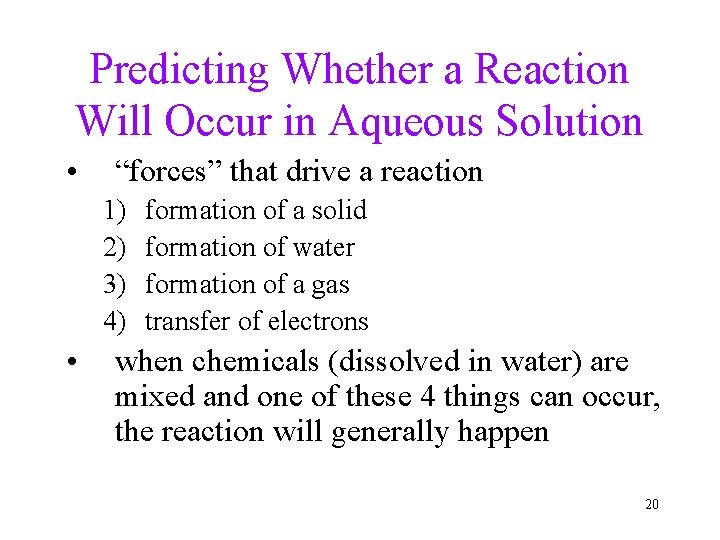Predicting Whether a Reaction Will Occur in Aqueous Solution • “forces” that drive a reaction 1) 2) 3) 4) • formation of a solid formation of water formation of a gas transfer of electrons when chemicals (dissolved in water) are mixed and one of these 4 things can occur, the reaction will generally happen 20Dissociation • when ionic compounds dissolve in water, the anions and cations are separated from each other - this is called dissociation ü however not all ionic compounds are soluble in water! • when compounds containing polyatomic ions dissociate, the polyatomic group stays together as one ion 21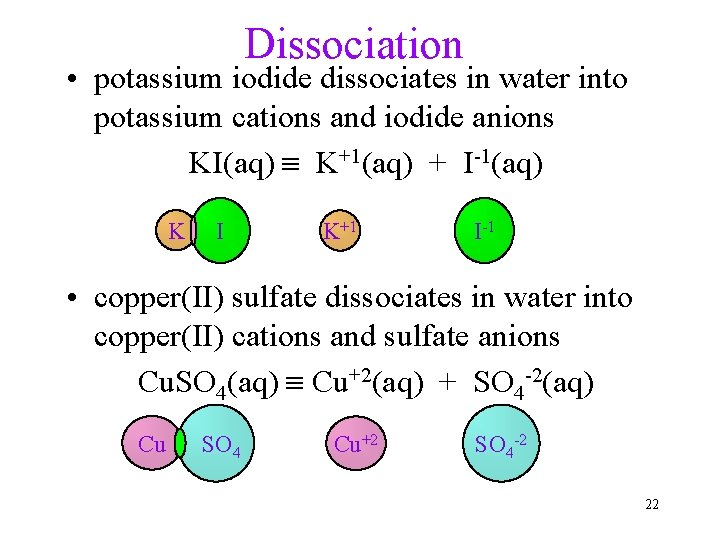Dissociation • potassium iodide dissociates in water into potassium cations and iodide anions KI(aq) K+1(aq) + I-1(aq) K I K+1 I-1 • copper(II) sulfate dissociates in water into copper(II) cations and sulfate anions Cu. SO 4(aq) Cu+2(aq) + SO 4 -2(aq) Cu SO 4 Cu+2 SO 4 -2 22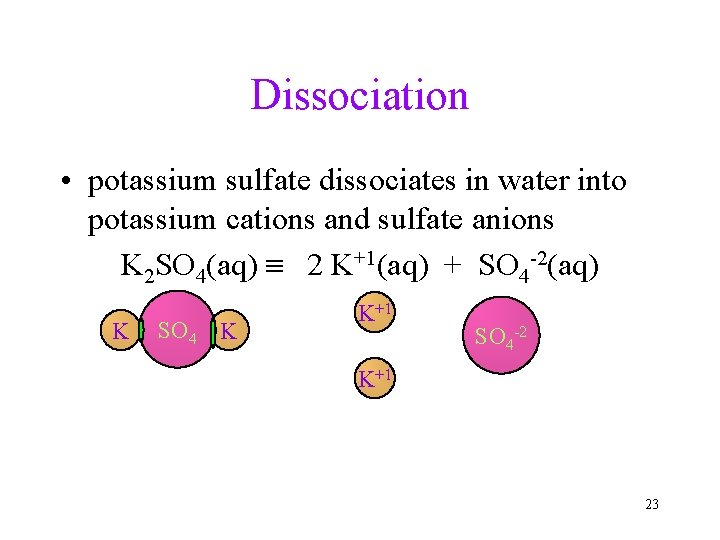Dissociation • potassium sulfate dissociates in water into potassium cations and sulfate anions K 2 SO 4(aq) 2 K+1(aq) + SO 4 -2(aq) K SO 4 K K+1 SO 4 -2 K+1 23Electrolytes • electrolytes are substances whose water solution is a conductor of electricity • all electrolyte have ions dissolved in water 24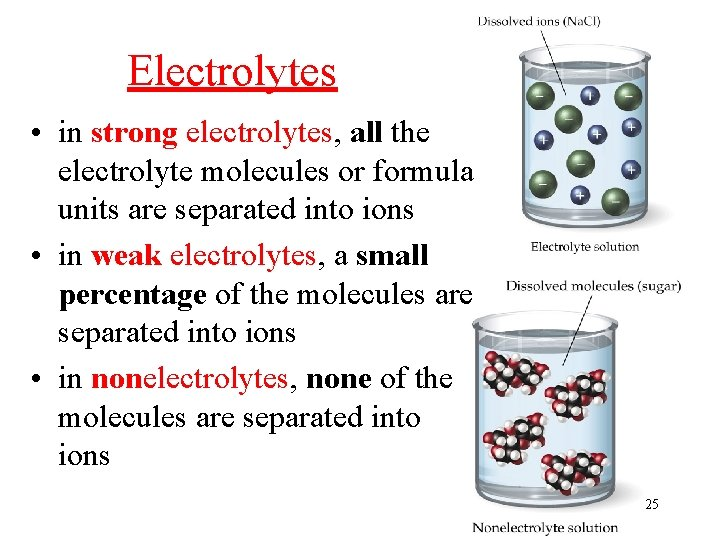Electrolytes • in strong electrolytes, all the electrolyte molecules or formula units are separated into ions • in weak electrolytes, a small percentage of the molecules are separated into ions • in nonelectrolytes, none of the molecules are separated into ions 25Types of Electrolytes • salts = water soluble ionic compounds üall strong electrolytes • acids = form H+1 ions in water solution üsour taste üreact and dissolve many metals ü strong acid = strong electrolyte, weak acid = weak electrolyte • bases = water soluble metal hydroxides übitter taste, slippery (soapy) feeling solutions üincreases the OH-1 concentration 26When will a Salt Dissolve? • a compound is soluble in a liquid if it dissolves in that liquid ü Na. Cl is soluble in water, but Ag. Cl is not • a compound is insoluble if a significant amount does not dissolve in that liquid ü Ag. Cl is insoluble in water Ø though there is a very small amount dissolved, but not enough to be significant 27When will a Salt Dissolve? • Predicting whether a compound will dissolve in water is not easy • The best way to do it is to do some experiments to test whether a compound will dissolve in water, then develop some rules based on those experimental results üwe call this method the empirical method 28Solubility Rules Compounds that are Generally Soluble in Water Compounds Containing the Exceptions following Ions are Generally (when combined with ions on the Soluble left the compound is insoluble) Li+, Na+, K+, NH 4+ none NO 3–, C 2 H 3 O 2– none Cl–, Br–, I– Ag+, Hg 22+, Pb 2+ SO 42– Ca 2+, Sr 2+, Ba 2+, Pb 2+ 29Solubility Rules Compounds that are Generally Insoluble Exceptions Compounds Containing the (when combined with ions on the following Ions are Generally left the compound is soluble or Insoluble slightly soluble) OH– S 2– CO 32–, PO 43– Li+, Na+, K+, NH 4+, Ca 2+, Sr 2+, Ba 2+ Li+, Na+, K+, NH 4+ 30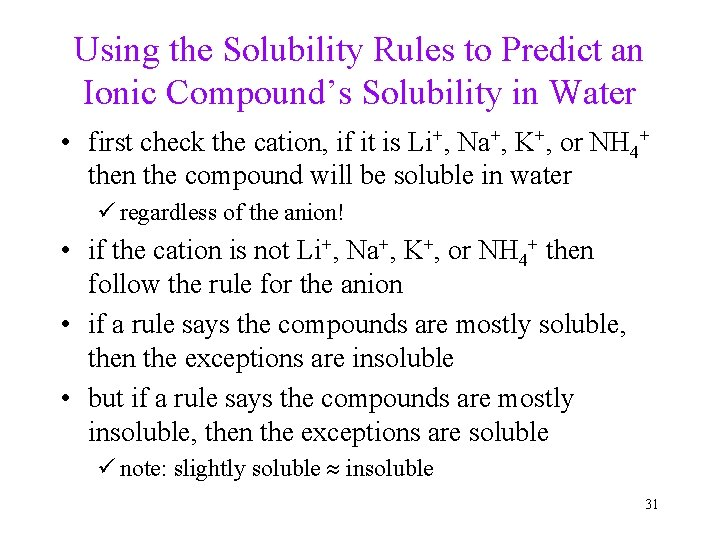Using the Solubility Rules to Predict an Ionic Compound’s Solubility in Water • first check the cation, if it is Li+, Na+, K+, or NH 4+ then the compound will be soluble in water ü regardless of the anion! • if the cation is not Li+, Na+, K+, or NH 4+ then follow the rule for the anion • if a rule says the compounds are mostly soluble, then the exceptions are insoluble • but if a rule says the compounds are mostly insoluble, then the exceptions are soluble ü note: slightly soluble insoluble 31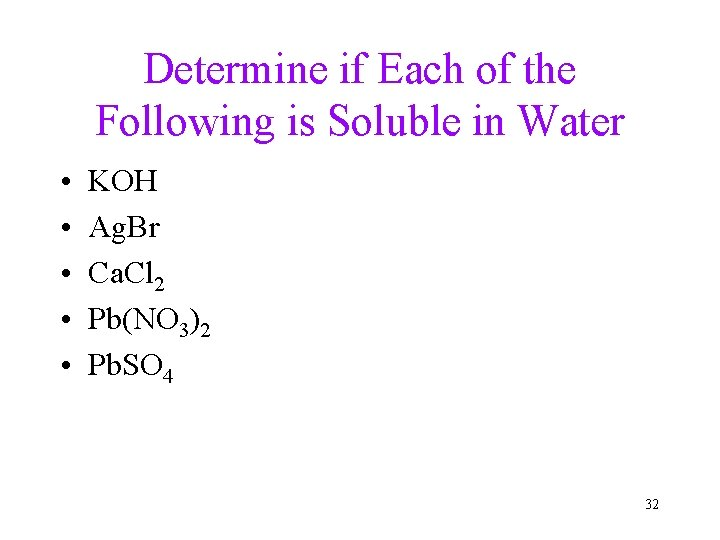Determine if Each of the Following is Soluble in Water • • • KOH Ag. Br Ca. Cl 2 Pb(NO 3)2 Pb. SO 4 32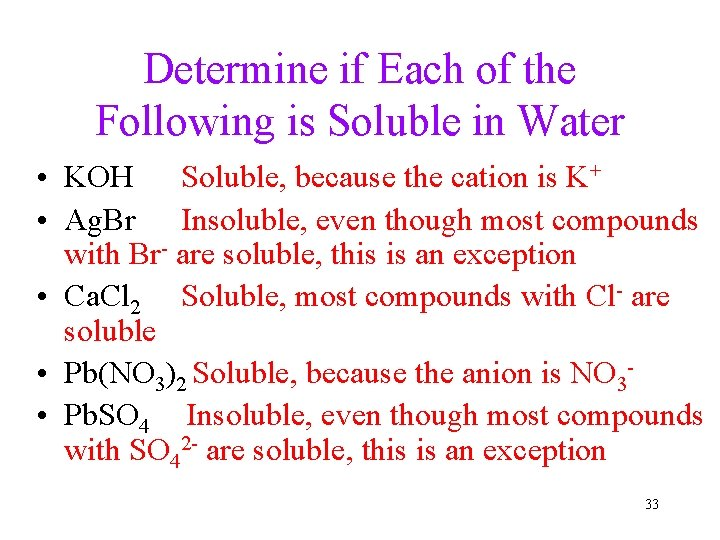Determine if Each of the Following is Soluble in Water • KOH Soluble, because the cation is K+ • Ag. Br Insoluble, even though most compounds with Br- are soluble, this is an exception • Ca. Cl 2 Soluble, most compounds with Cl- are soluble • Pb(NO 3)2 Soluble, because the anion is NO 3 • Pb. SO 4 Insoluble, even though most compounds with SO 42 - are soluble, this is an exception 33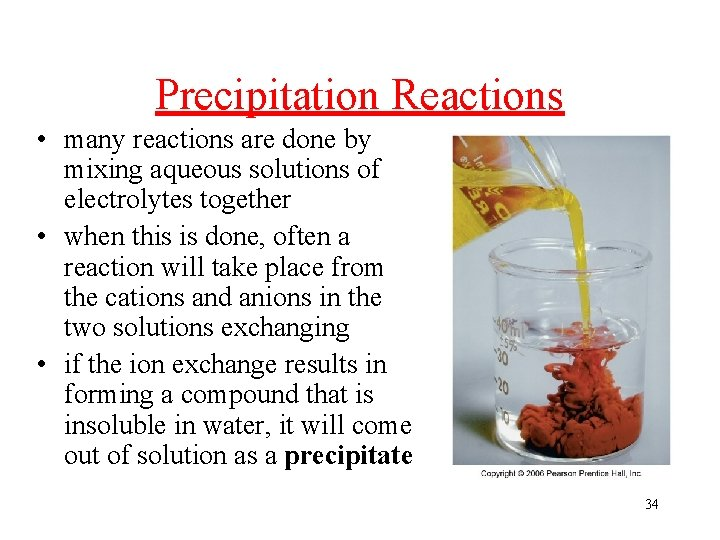Precipitation Reactions • many reactions are done by mixing aqueous solutions of electrolytes together • when this is done, often a reaction will take place from the cations and anions in the two solutions exchanging • if the ion exchange results in forming a compound that is insoluble in water, it will come out of solution as a precipitate 34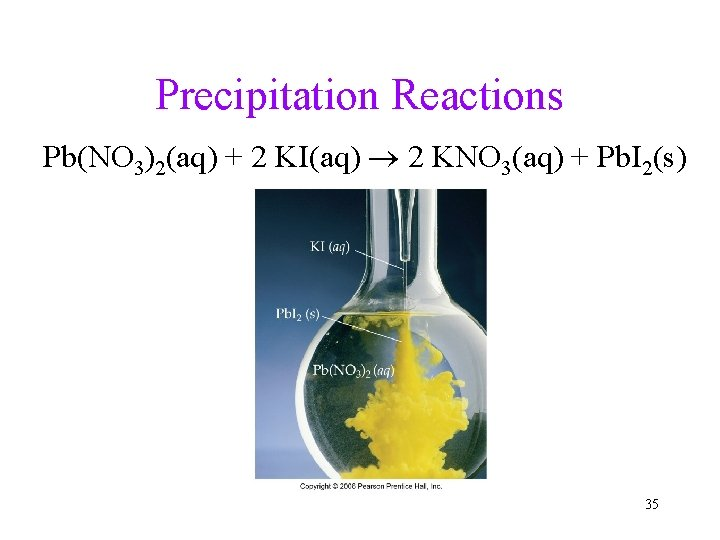Precipitation Reactions Pb(NO 3)2(aq) + 2 KI(aq) 2 KNO 3(aq) + Pb. I 2(s) 35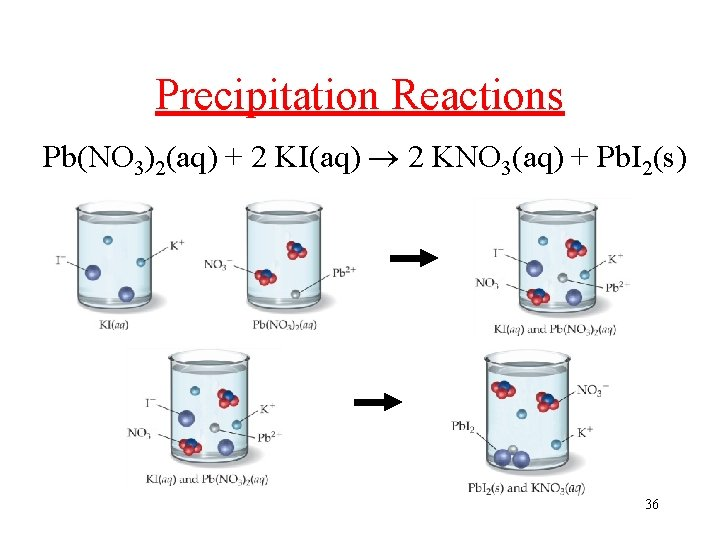Precipitation Reactions Pb(NO 3)2(aq) + 2 KI(aq) 2 KNO 3(aq) + Pb. I 2(s) 36No Precipitate Formation = No Reaction KI(aq) + Na. Cl(aq) KCl(aq) + Na. I(aq) all ions still present, no reaction 37Process for Predicting the Products of a Precipitation Reaction 1. Determine what ions each aqueous reactant has 2. Exchange Ions ü (+) ion from one reactant with (-) ion from other 3. Balance Charges of combined ions to get formula of each product 4. Balance the Equation ü count atoms 5. Determine Solubility of Each Product in Water ü use the Solubility Rules ü if product is insoluble or slightly soluble, it will precipitate ü if neither product will precipitate, no reaction 38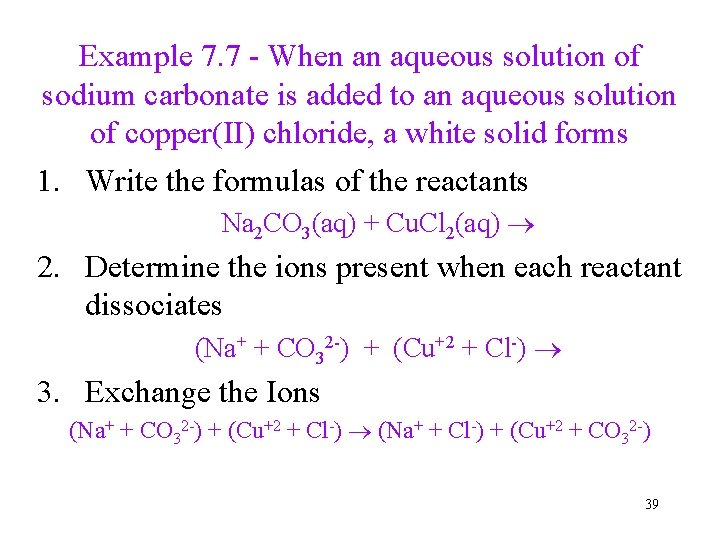Example 7. 7 - When an aqueous solution of sodium carbonate is added to an aqueous solution of copper(II) chloride, a white solid forms 1. Write the formulas of the reactants Na 2 CO 3(aq) + Cu. Cl 2(aq) 2. Determine the ions present when each reactant dissociates (Na+ + CO 32 -) + (Cu+2 + Cl-) 3. Exchange the Ions (Na+ + CO 32 -) + (Cu+2 + Cl-) (Na+ + Cl-) + (Cu+2 + CO 32 -) 39Example 7. 7 - When an aqueous solution of sodium carbonate is added to an aqueous solution of copper(II) chloride, a white solid forms 4. Write the formulas of the products ü cross charges and reduce Na 2 CO 3(aq) + Cu. Cl 2(aq) Na. Cl + Cu. CO 3 5. Balance the Equation Na 2 CO 3(aq) + Cu. Cl 2(aq) 2 Na. Cl + Cu. CO 3 40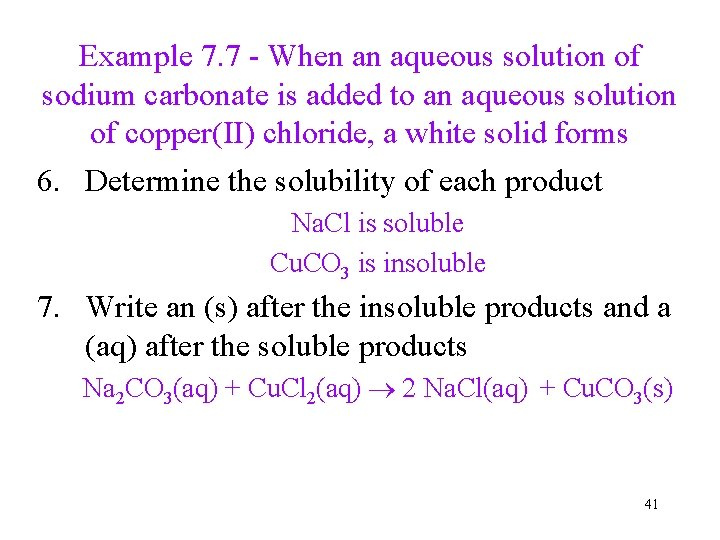Example 7. 7 - When an aqueous solution of sodium carbonate is added to an aqueous solution of copper(II) chloride, a white solid forms 6. Determine the solubility of each product Na. Cl is soluble Cu. CO 3 is insoluble 7. Write an (s) after the insoluble products and a (aq) after the soluble products Na 2 CO 3(aq) + Cu. Cl 2(aq) 2 Na. Cl(aq) + Cu. CO 3(s) 41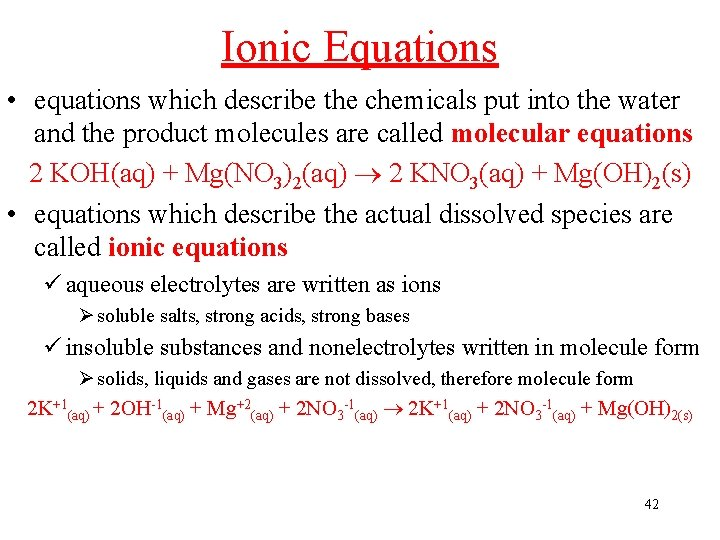Ionic Equations • equations which describe the chemicals put into the water and the product molecules are called molecular equations 2 KOH(aq) + Mg(NO 3)2(aq) 2 KNO 3(aq) + Mg(OH)2(s) • equations which describe the actual dissolved species are called ionic equations ü aqueous electrolytes are written as ions Ø soluble salts, strong acids, strong bases ü insoluble substances and nonelectrolytes written in molecule form Ø solids, liquids and gases are not dissolved, therefore molecule form 2 K+1(aq) + 2 OH-1(aq) + Mg+2(aq) + 2 NO 3 -1(aq) 2 K+1(aq) + 2 NO 3 -1(aq) + Mg(OH)2(s) 42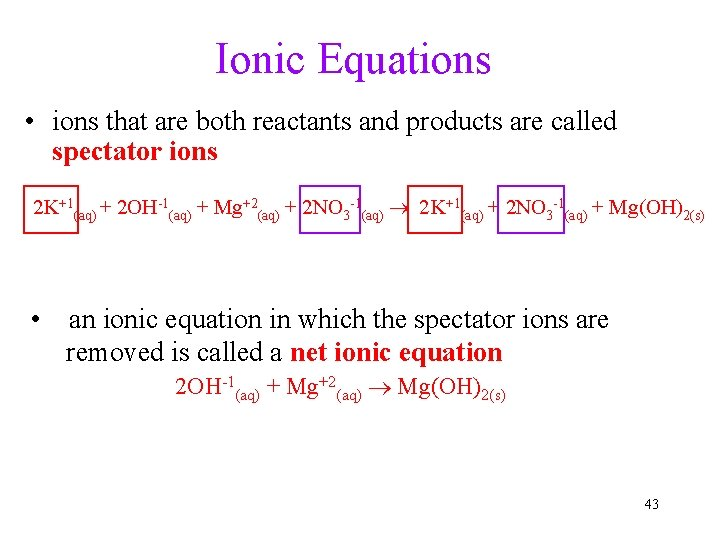Ionic Equations • ions that are both reactants and products are called spectator ions 2 K+1(aq) + 2 OH-1(aq) + Mg+2(aq) + 2 NO 3 -1(aq) 2 K+1(aq) + 2 NO 3 -1(aq) + Mg(OH)2(s) • an ionic equation in which the spectator ions are removed is called a net ionic equation 2 OH-1(aq) + Mg+2(aq) Mg(OH)2(s) 43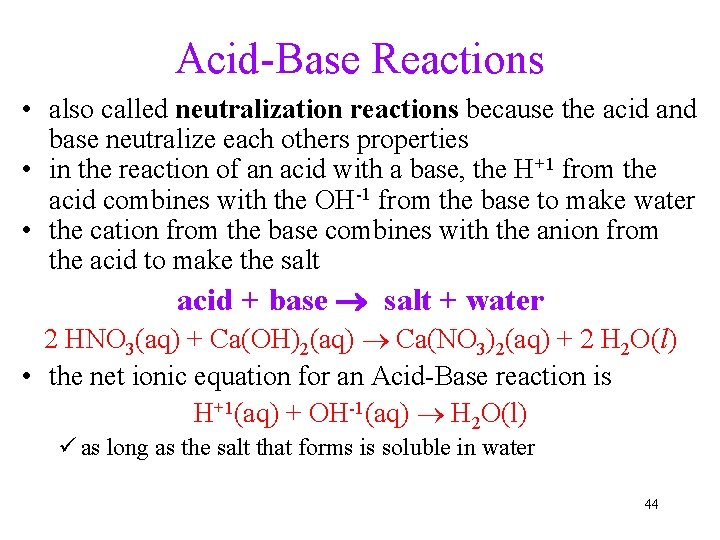Acid-Base Reactions • also called neutralization reactions because the acid and base neutralize each others properties • in the reaction of an acid with a base, the H+1 from the acid combines with the OH-1 from the base to make water • the cation from the base combines with the anion from the acid to make the salt acid + base salt + water 2 HNO 3(aq) + Ca(OH)2(aq) Ca(NO 3)2(aq) + 2 H 2 O(l) • the net ionic equation for an Acid-Base reaction is H+1(aq) + OH-1(aq) H 2 O(l) ü as long as the salt that forms is soluble in water 44Example 7. 11 - Write the molecular, ionic and net -ionic equation for the reaction of aqueous nitric acid with aqueous calcium hydroxide 1. Write the formulas of the reactants HNO 3(aq) + Ca(OH)2(aq) 2. Determine the ions present when each reactant dissociates (H+ + NO 3 -) + (Ca+2 + OH-) 3. Exchange the ions, H+1 combines with OH-1 to make H 2 O(l) (H+ + NO 3 -) + (Ca+2 + OH-) (Ca+2 + NO 3 -) + H 2 O(l) 45Example 7. 11 - Write the molecular, ionic and net -ionic equation for the reaction of aqueous nitric acid with aqueous calcium hydroxide 4. Write the formulas of the products ü cross charges and reduce HNO 3(aq) + Ca(OH)2(aq) Ca(NO 3)2 + H 2 O(l) 5. Balance the Equation ü may be quickly balanced by matching the numbers of H and OH to make H 2 O ü coefficient of the salt is always 1 2 HNO 3(aq) + Ca(OH)2(aq) Ca(NO 3)2 + 2 H 2 O(l) 46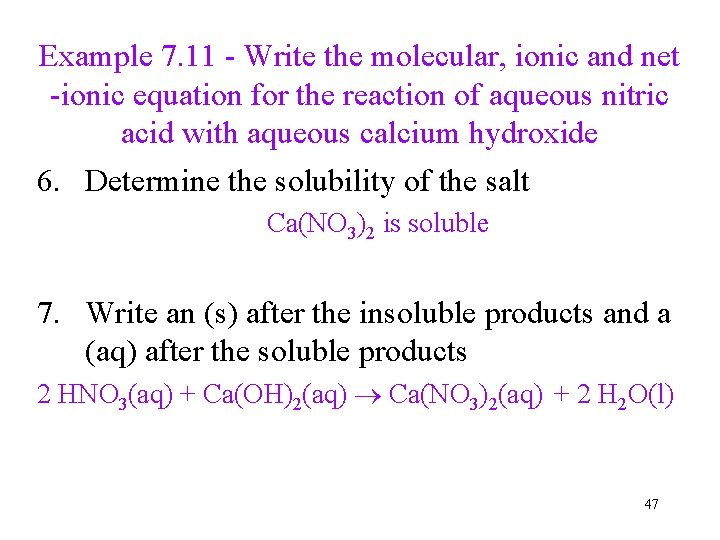Example 7. 11 - Write the molecular, ionic and net -ionic equation for the reaction of aqueous nitric acid with aqueous calcium hydroxide 6. Determine the solubility of the salt Ca(NO 3)2 is soluble 7. Write an (s) after the insoluble products and a (aq) after the soluble products 2 HNO 3(aq) + Ca(OH)2(aq) Ca(NO 3)2(aq) + 2 H 2 O(l) 47Example 7. 11 - Write the molecular, ionic and net-ionic equation for the reaction of aqueous nitric acid with aqueous calcium hydroxide 8. Dissociate all aqueous materials to get complete ionic equation ü not H 2 O 2 H+(aq) + 2 NO 3 -(aq) + Ca+2(aq) + 2 OH-(aq) Ca+2(aq) + 2 NO 3 -(aq) + H 2 O(l) 9. Eliminate spectator ions to get net-ionic equation 2 H+1(aq) + 2 OH-1(aq) 2 H 2 O(l) H+1(aq) + OH-1(aq) H 2 O(l) 48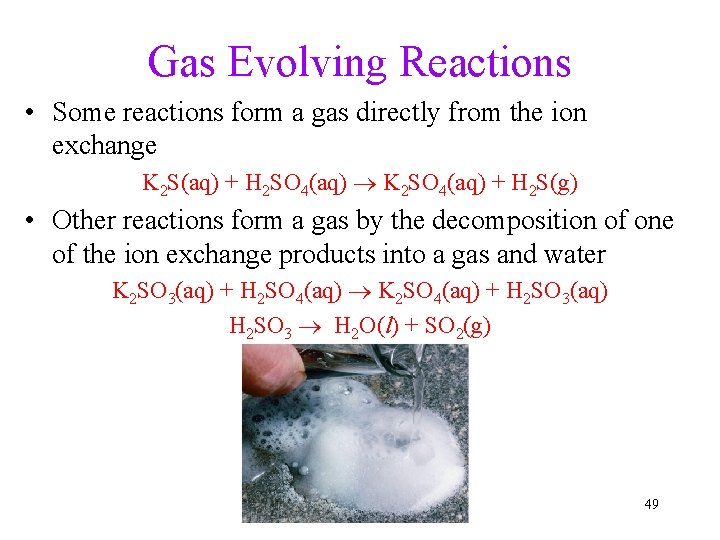Gas Evolving Reactions • Some reactions form a gas directly from the ion exchange K 2 S(aq) + H 2 SO 4(aq) K 2 SO 4(aq) + H 2 S(g) • Other reactions form a gas by the decomposition of one of the ion exchange products into a gas and water K 2 SO 3(aq) + H 2 SO 4(aq) K 2 SO 4(aq) + H 2 SO 3(aq) H 2 SO 3 H 2 O(l) + SO 2(g) 49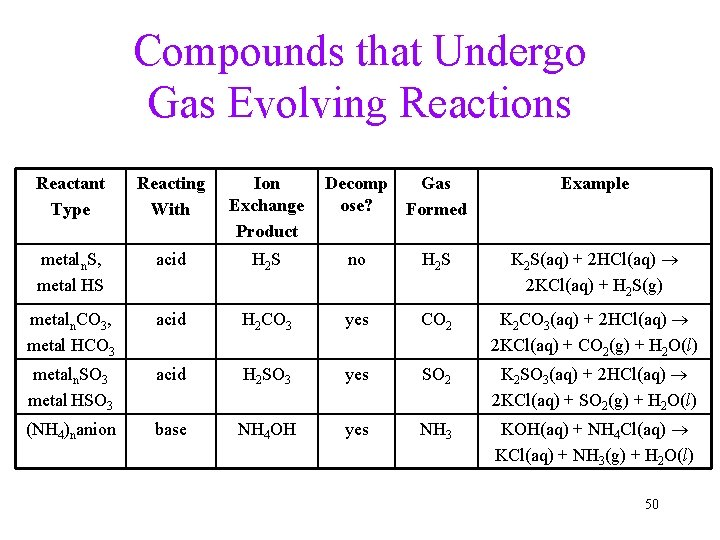Compounds that Undergo Gas Evolving Reactions Reactant Type Reacting With Ion Exchange Product Decomp Gas ose? Formed Example metaln. S, metal HS acid H 2 S no H 2 S K 2 S(aq) + 2 HCl(aq) 2 KCl(aq) + H 2 S(g) metaln. CO 3, metal HCO 3 acid H 2 CO 3 yes CO 2 K 2 CO 3(aq) + 2 HCl(aq) 2 KCl(aq) + CO 2(g) + H 2 O(l) metaln. SO 3 metal HSO 3 acid H 2 SO 3 yes SO 2 K 2 SO 3(aq) + 2 HCl(aq) 2 KCl(aq) + SO 2(g) + H 2 O(l) (NH 4)nanion base NH 4 OH yes NH 3 KOH(aq) + NH 4 Cl(aq) KCl(aq) + NH 3(g) + H 2 O(l) 50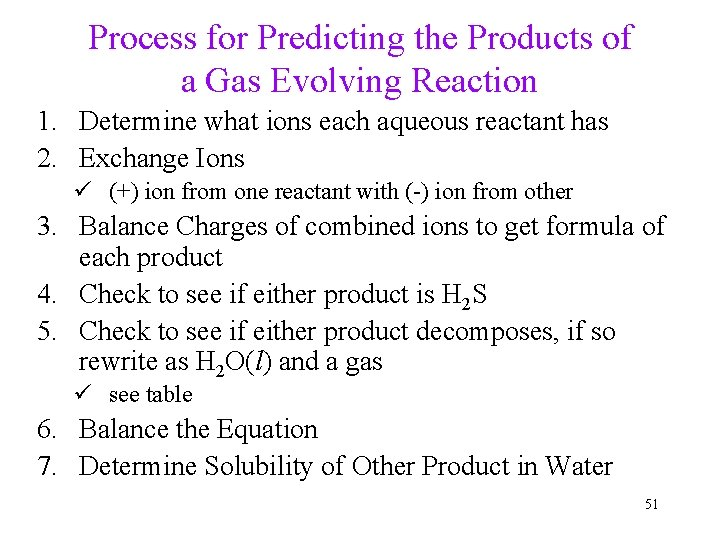Process for Predicting the Products of a Gas Evolving Reaction 1. Determine what ions each aqueous reactant has 2. Exchange Ions ü (+) ion from one reactant with (-) ion from other 3. Balance Charges of combined ions to get formula of each product 4. Check to see if either product is H 2 S 5. Check to see if either product decomposes, if so rewrite as H 2 O(l) and a gas ü see table 6. Balance the Equation 7. Determine Solubility of Other Product in Water 51Example - When an aqueous solution of sodium sulfite is added to an aqueous solution of nitric acid, a gas evolves 1. Write the formulas of the reactants Na 2 SO 3(aq) + HNO 3(aq) 2. Determine the ions present when each reactant dissociates (Na+1 + SO 3 -2) + (H+1 + NO 3 -1) 3. Exchange the Ions (Na+1 + SO 3 -2) + (H+1 + NO 3 -1) (Na+1 + NO 3 -1) + (H+1 + SO 3 -2) 52Example - When an aqueous solution of sodium sulfite is added to an aqueous solution of nitric acid, a gas evolves 4. Write the formulas of the products ü cross charges and reduce Na 2 SO 3(aq) + HNO 3(aq) Na. NO 3 + H 2 SO 3 5. Check to see either product H 2 S - No 6. Check to see of either product decomposes – Yes ü H 2 SO 3 decomposes into SO 2(g) + H 2 O(l) Na 2 SO 3(aq) + HNO 3(aq) Na. NO 3 + SO 2(g) + H 2 O(l) 53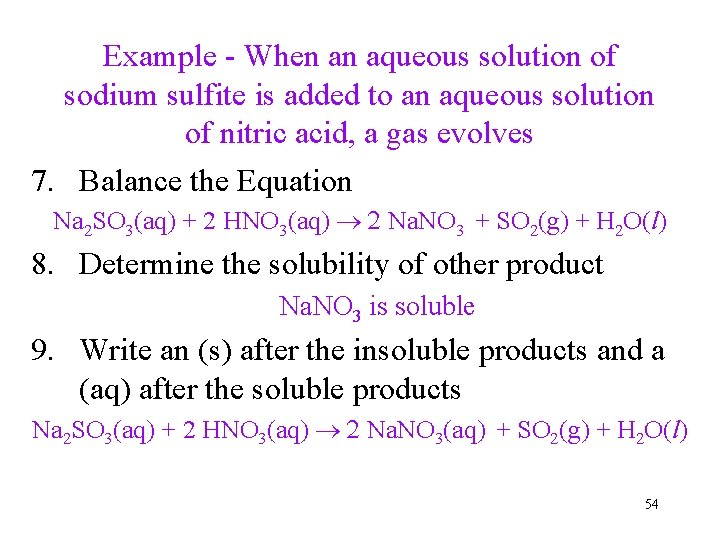Example - When an aqueous solution of sodium sulfite is added to an aqueous solution of nitric acid, a gas evolves 7. Balance the Equation Na 2 SO 3(aq) + 2 HNO 3(aq) 2 Na. NO 3 + SO 2(g) + H 2 O(l) 8. Determine the solubility of other product Na. NO 3 is soluble 9. Write an (s) after the insoluble products and a (aq) after the soluble products Na 2 SO 3(aq) + 2 HNO 3(aq) 2 Na. NO 3(aq) + SO 2(g) + H 2 O(l) 54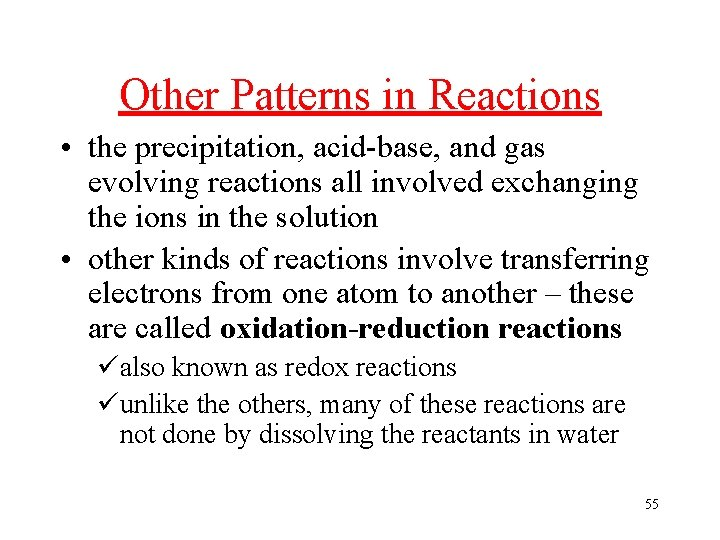Other Patterns in Reactions • the precipitation, acid-base, and gas evolving reactions all involved exchanging the ions in the solution • other kinds of reactions involve transferring electrons from one atom to another – these are called oxidation-reduction reactions üalso known as redox reactions üunlike the others, many of these reactions are not done by dissolving the reactants in water 55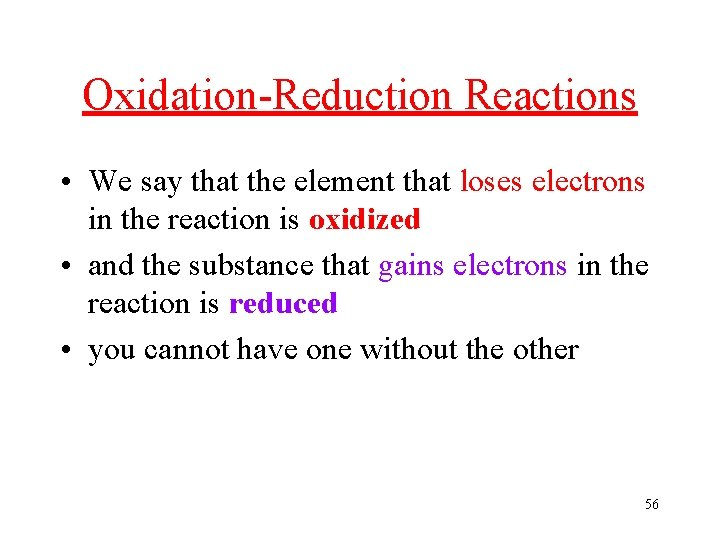Oxidation-Reduction Reactions • We say that the element that loses electrons in the reaction is oxidized • and the substance that gains electrons in the reaction is reduced • you cannot have one without the other 56Reactions of Metals with Nonmetals (Oxidation-Reduction) • metals react with nonmetals to form ionic compounds ü ionic compounds are solids at room temperature • the metal loses electrons and becomes a cation ü the metal undergoes oxidation • the nonmetal gains electrons and becomes an anion ü the nonmetal undergoes reduction • In the reaction, electrons are transferred from the metal to the nonmetal 2 Na(s) + Cl 2(g) Na. Cl(s) 57Oxidation-Reduction Reactions • any reaction that has an element that is uncombined on one side and combined on the other is a redox reaction üuncombined = free element ü 2 CO + O 2 2 CO 2 ü 2 N 2 O 5 4 NO 2 + O 2 ü 3 C + Fe 2 O 3 3 CO + 2 Fe üMg + Cl 2 Mg. Cl 2 • any reaction where a cation changes charge is redox üCu. Cl + Fe. Cl 3 Fe. Cl 2 + Cu. Cl 2 üSn. Cl 2 + F 2 Sn. Cl 2 F 2 58Combustion Reactions • Reactions in which O 2(g) is a reactant are called Combustion Reactions • Combustion reactions release lots of energy • Combustion reactions are a subclass of Oxidation. Reduction reactions 2 C 8 H 18(g) + 25 O 2(g) 16 CO 2(g) + 18 H 2 O(g) 59Combustion Products • to predict the products of a combustion reaction, combine each element in the other reactant with oxygen Reactant Combustion Product contains C CO 2(g) contains H H 2 O(g) contains S SO 2(g) contains N NO(g) or NO 2(g) contains metal M 2 On(s) 60Classifying Reactions • one way is based on the process that happens üprecipitation, neutralization, formation of a gas, or transfer of electrons 61Classifying Reactions • another scheme classifies reactions by what the atoms do Type of Reaction Synthesis General Equation A + B AB Decomposition AB A + B Displacement A + BC AC + B Double Displacement AB + CD AD + CB 62Synthesis Reactions • also known as Composition or Combination reactions • two (or more) reactants combine together to make one product üsimpler substances combining together 2 CO + O 2 2 CO 2 2 Mg + O 2 2 Mg. O Hg. I 2 + 2 KI K 2 Hg. I 4 63Decomposition Reactions • a large molecule is broken apart into smaller molecules or its elements ücaused by addition of energy into the molecule • have only one reactant, make 2 or more products 64Decomposition of Water 65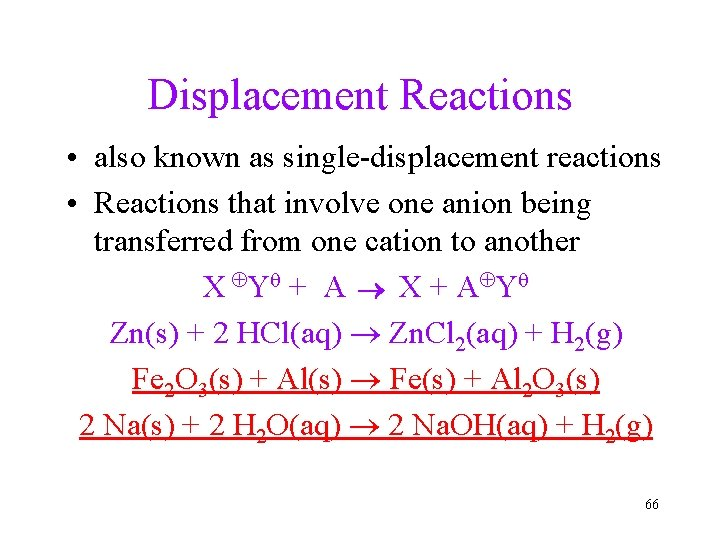Displacement Reactions • also known as single-displacement reactions • Reactions that involve one anion being transferred from one cation to another X ÅY q + A X + A ÅY q Zn(s) + 2 HCl(aq) Zn. Cl 2(aq) + H 2(g) Fe 2 O 3(s) + Al(s) Fe(s) + Al 2 O 3(s) 2 Na(s) + 2 H 2 O(aq) 2 Na. OH(aq) + H 2(g) 66Displacement of Copper by Zinc 67Double Displacement Reactions • two ionic compounds exchange ions • may be followed by decomposition of one of the products to make a gas • X ÅYq (aq) + A ÅBq (aq) XB + AY • precipitation, acid-base and gas-evolving reactions are also double displacement reactions 68